# 《TCP IP 详解卷1：协议》阅读笔记 - 第五章

+关注继续查看

IP是TCP/IP协议族中的核心协议，TCP、UDP、ICMP、IGMP数据都通过IP数据报传输。IP提供了一种"尽力而为、无连接"的数据交付服务：尽力而为表示不保证IP数据报能成功到达目的地；无连接意味着IP不维护网络单元中数据报相关的任何链接状态信息，每个数据报独立于其他数据报来处理，这也意味着IP数据报的可不按顺序交付。

IPv4头部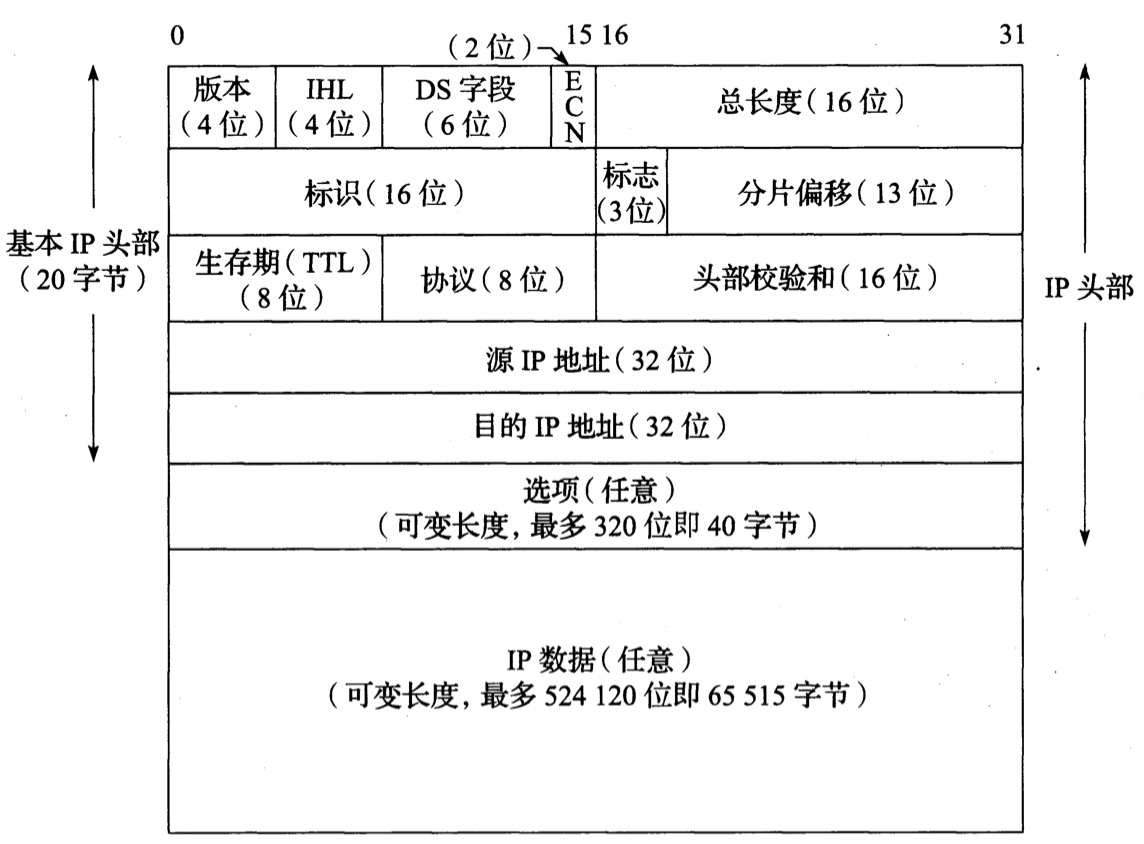1. 版本标识(IPv4为4，IPv6为6)；

2. IHL(Internet头部长度)；

3. DC字段(服务类型字段)，指定一个等效的通信类型字段，前6位是区分服务字段，后2位(ECN)是显式拥塞通知字段或指示位；

4. 总长度，IPv4数据报的总长度，由于它是一个16位字段，所以可以算出IPv4数据报的最大长度是65535字节(理想化的最大，实际通信由多方因素计算后取最小值)；

5. 标识字段，帮助标识由IPv4主机发送的数据报，避免数据报分片混淆；标志是一个3位的控制字段，表示是否允许数据分片、该数据报是否是末尾的数据报；分片偏移字段给出该分片负载字节中的第一个字节在原始IPv4数据中的偏移量；

6. TTL(生存期)用于设置一个数据报可经过的路由器数量上限，每经过一台路由器，该值减1，直至0，用于防止由于出现路由环路而导致的数据报循环；

7. 协议字段表示数据报有效载荷部分的数据类型，最常用值为17(UDP)和6(TCP)；

8. 头部校验和字段仅计算IPv4头部，不检查数据报有效载荷的正确性；

9. 源IP地址和目标IP地址，数据通信的两端地址。

IPv4支持一些可供数据报选择的选项，填充在选项字段，选项有一个8位的类型字段标识：复制(1位)、类别(2位)、编号(5位)。

IPv4选项如下表：IPv6头部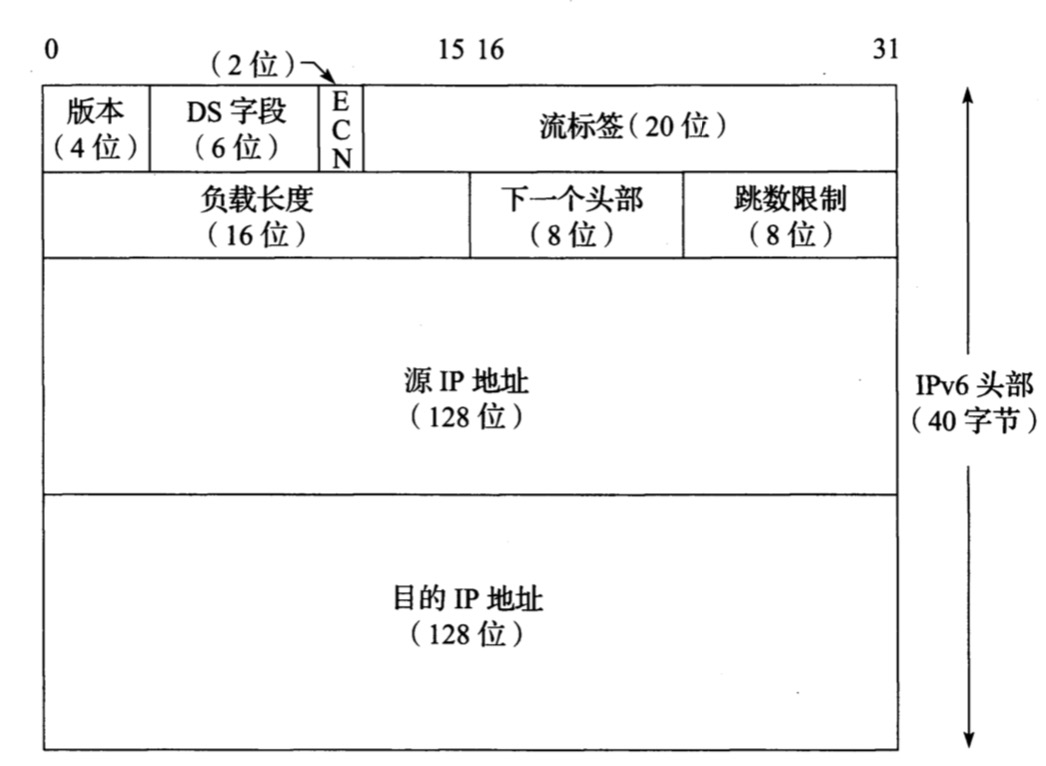1. 流标签以标识同一个流里面的报文；

2. 负载长度(对比标识字段)字段提供IPv6数据报的长度，不包括头部长度，但包括IPv6扩展头部；、

3. 下一个头部字段用来指明报头后接的报文头部的类型，若存在扩展头，表示第一个扩展头的类型，否则表示其上层协议的类型，它是IPv6各种功能的核心实现方法；

4. 跳数限制(对比IPv4的TTL)

IPv6扩展头部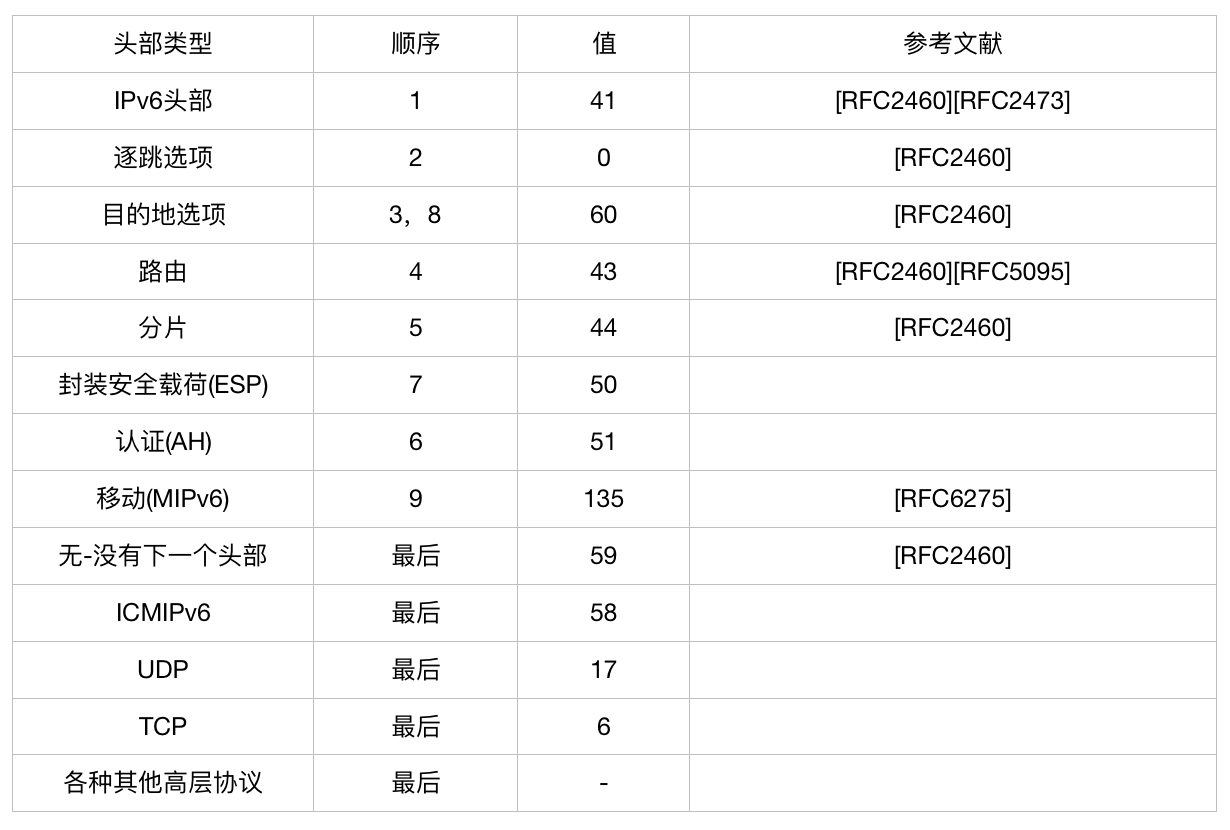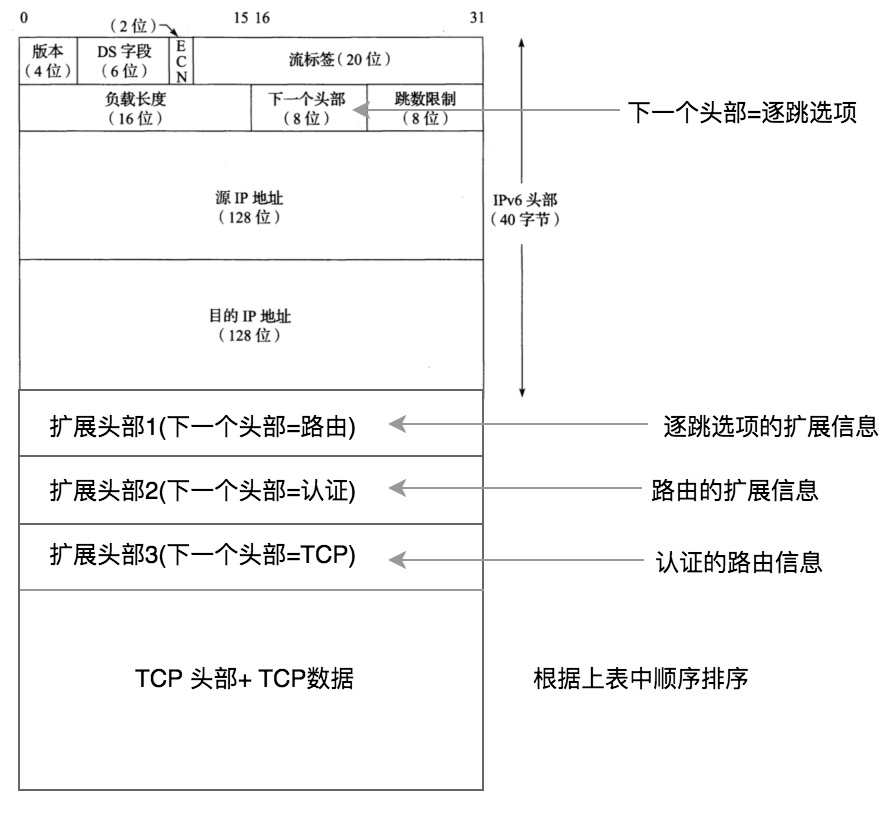IPv6选项

1. 填充1和填充N：填充1选项(类型0)是唯一缺少长度字段和值字段的选项。它仅有1字节长，取值为0。填充N选项(类型1)向头部的选项区域填充2字节或更多字节，对于n个填充字节，选项数据长度字段包含的值为(n-2)。

2. IPv6超大有效载荷：IPv6超大有效载荷选项指定了一种有效载荷大于65535字节的IPv6数据报，称为超大报文。超大有效载荷选项提供了一个32位的字段，用于携带有效载荷在65535~4294967295字节直接的数据报，当使用超大报文时，正常的敷在长度字段被设置位0。

3. 隧道封装限制：路由器想要将IPv6数据封装在一条隧道中，它首先检查隧道封装限制选项是否存在并置位，如果不为0，则可进行隧道封装，且新封装的IPv6数据报必须包括这个选项，其值相对之前的封装限制值减1，依次封装递减。

4. 路由器警告：与IPv4的该选项一样，指出包含需要路由器处理的信息。

5. 快速启动：适用于IPv4和IPv6，但目前建议仅用于专用网络。选项包括发送者需要的以比特/秒为单位的传输速率的编码值、QS TTL值和一些额外信息。

6. CALIPSO：于在某些专用网络中支持通用体系结构标签IPv6安全选项。它提供了一种为数据报做标记的方法，包括一个安全级别标识符和一些额外的信息。

7. 家乡地址：当使用IPV6移动选项时，这个选项保存发送数据报的IPv6节点的"家乡"地址。当远离"家乡"(典型位置的地址前缀)漫游时，通常为该节点分配一个不同的IP地址。该选项允许这个节点提供自已正常的家乡地址，以及它在漫游时的新地址(通常是临时分配)。当其他IPv6节点需要与移动节点通信时，它可以使用该节点的家乡地址。如果家乡地址选项存在，包含它的目的地选项头部必须出现在路由头部之后，并在分片和ESP头部之前。

"家乡"的概念来自典型位置的地址前缀，其设计目的是为了让移动设备用户，能够从一个网上系统中，移动到另一个网上系统，但是设备的IP地址保持不变。这能够使移动节点在移动中保持其连接性，实现跨越不同网段的漫游功能。

Internet校验和

Intenret校验和是一个被校验数据(如果被计算的字节数为奇数，用0填充)的16位反码和的反码，它能以相当高的概率确定接收的消息或其中的部内容是否与发送的相匹配。如果被计算数据包括一个校验和字段，该字段在进行校验和运算之前被设置为0，然后将计算出的校验和填充到该字段。为了检查一个包含校验和字段(头部、有效载荷等)的数据输入是否有效，需要对整个数据块(包括校验和字段)同样计算校验和。由于校验和字段本质上是其余数据校验和的反码，对正确接收的数据计算校验和应产生一个值0。

# 发送

# 接收

~(消息 + 校验和) = 0000

IPv6路由头部位发送方提供一种IPv6数据报控制机制，以控制数据报通过网络的路径。路由头部包含一个8位的路由类型标识符和一个8位的剩余部分字段。路由类型标识符为0或者2，分别代表类型0(RH0)和类型2(RH2)。剩余部分字段指出还有多少段路由需要处理，也就是在到达最终目的地之前仍需访问的中间节点数。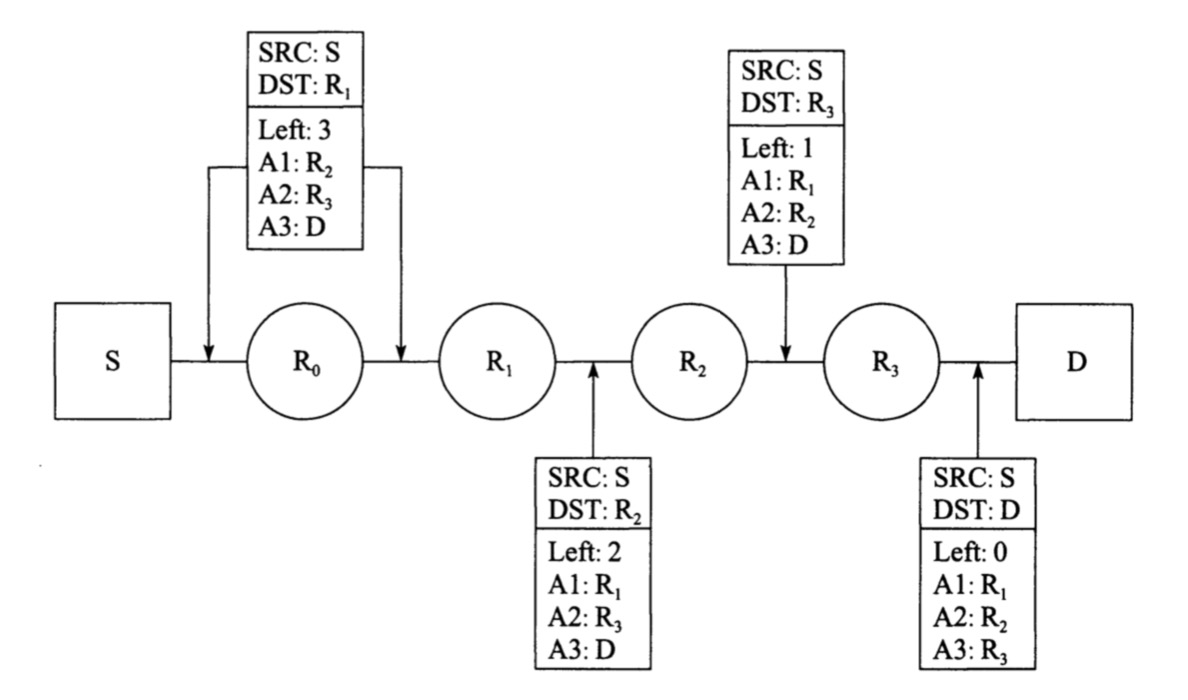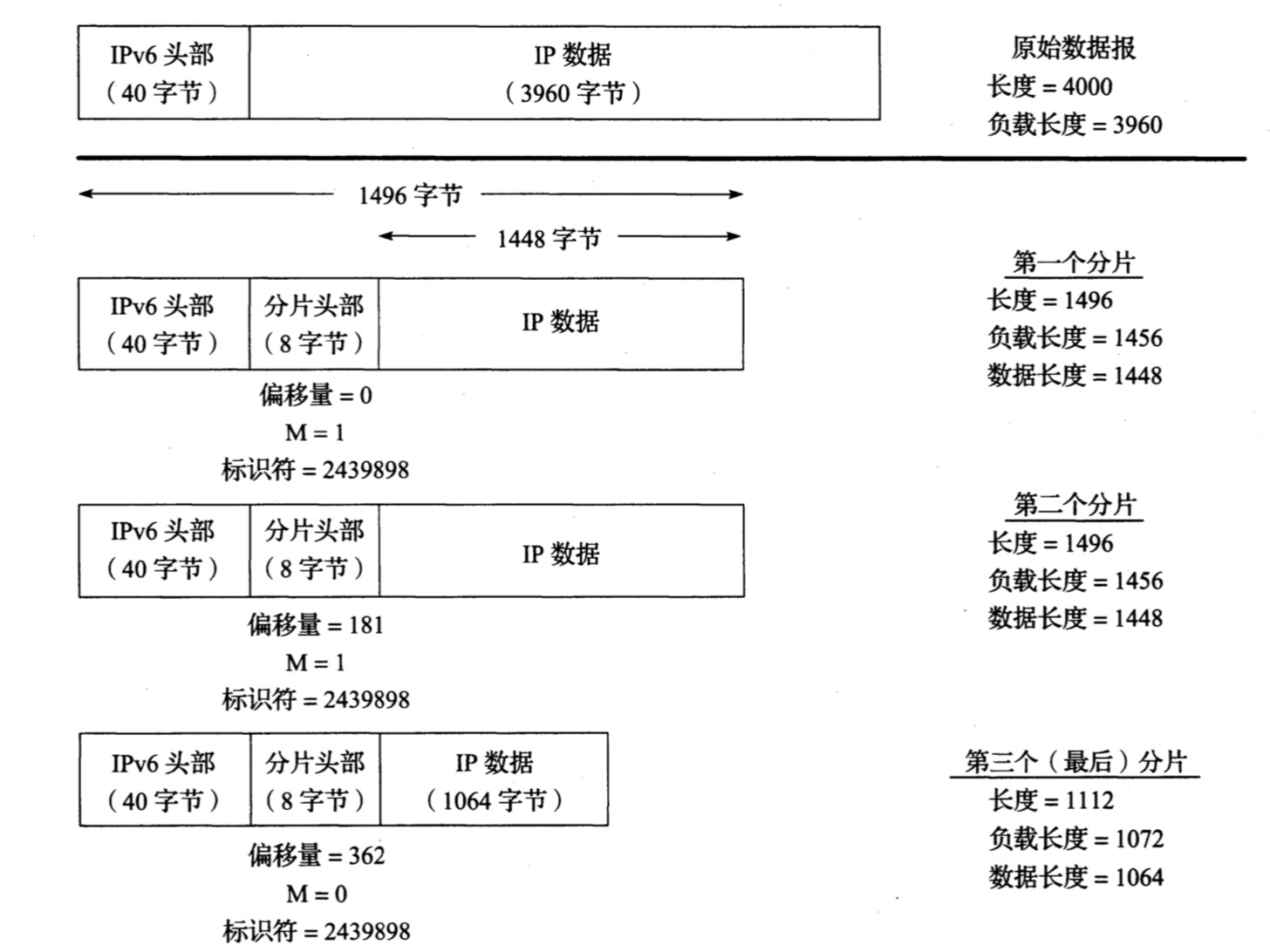1个3960字节的有效载荷被分为3个1448字节或更小的分片，每个分片包含一个带相同的标识符字段的分片头部，除了最后一个分片，所有分片的更多分片(M)字段设置为1。偏移量以8字节为单位，例如最后一个分片包含的数据是从原始数据开始处偏移(362*8)=2896字节。

IP转发

IP转发表包含以下信息：

1. 目的地：32位字段，,用于与一个掩码操作结果相匹配。用于发送到所有目的地的默认路由情况下，地址全设为0。

2. 掩码：32位字段，用作数据报目的IP地址按位与操作的掩码。

3. 下一跳：下一个IP实体的32位IPv4地址或者128位的IPv6地址，数据报将被转发到这。

4. 接口：它包含一个由IP层使用的标识符，以确定将数据报发送到下一跳的网络接口。

IP转发逐跳进行，当一台主机或路由器中的IP层需要向下一跳的路由器或主机发送一个数据报时，它首先检查数据报中的目的IP地址(D)。在转发表中使用该值D来执行最长前缀匹配算法：在表中搜索具有以下属性的所有条目：(D^mj)=dj。

1. 其中mj是索引为j的转发条目ej的掩码字段值，dj是转发条目ej的目的字段值。

2. 这意味着目的IP地址D与每个转发表条目中的掩码mj执行按位与，并将该结果与同一个条目中的目的地dj比较。

3. 如果满足这个属性，该条目ej与目的IP地址进行"匹配"。

4. 当进行匹配时，该算法查看这个条目的索引j，以及在掩码mj中有多少位设置为1。设置为1的位数越多，说明匹配的越好。

5. 选择最匹配的条目ek(即掩码mk中最多位为1的条目)，将其下一跳字段nk作为转发数据报的下一跳IP地址。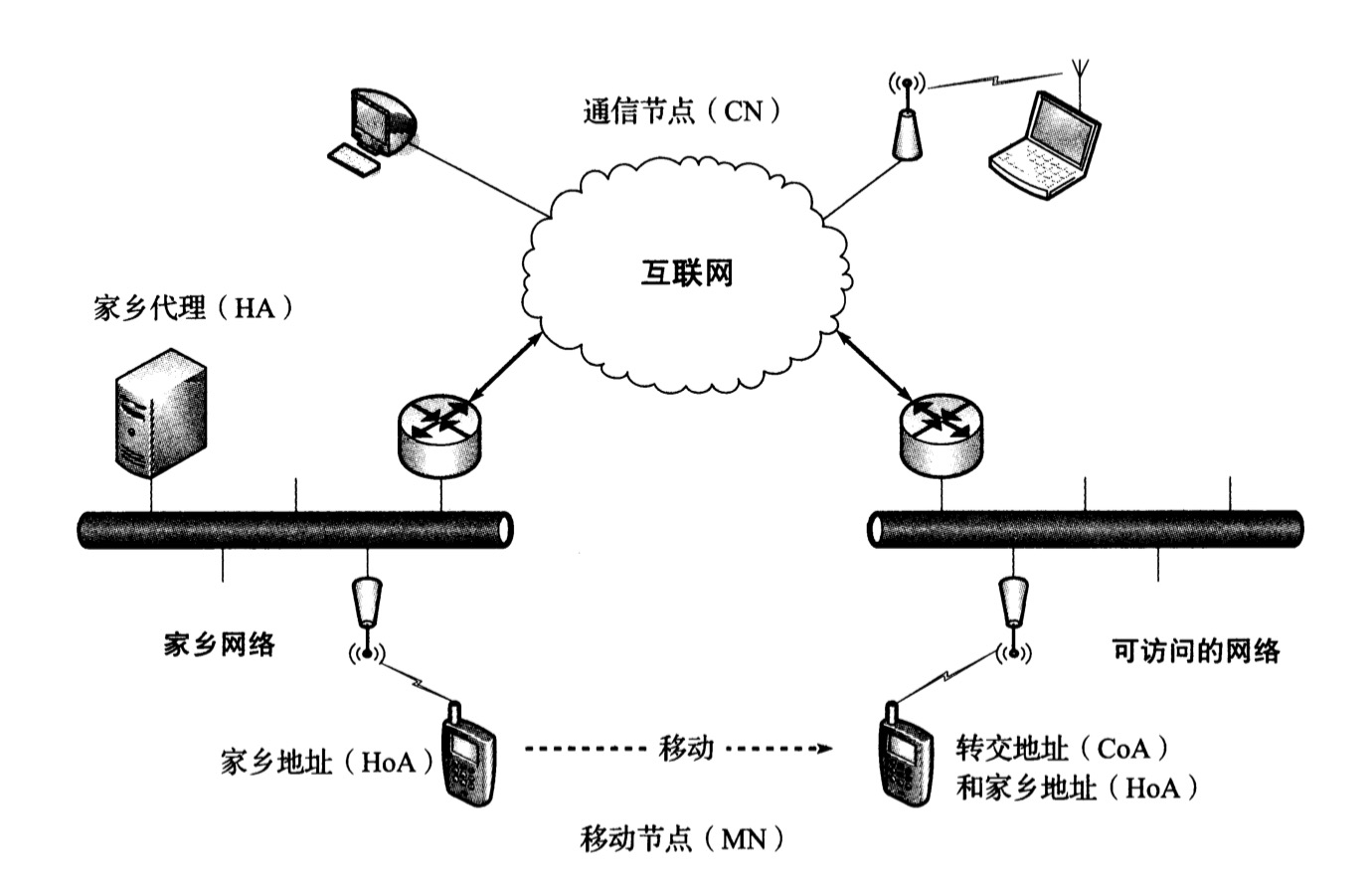1. 一台可能移动的主句称为移动节点(MN)，与它通信的主句称为通信节点(CN)。

2. MN被赋予一个由家乡网络的网络前缀获得的IP地址，被称为家乡地址HoA。

3. 当它漫游到一个可访问的网络时，它被赋予另外一个地址，称为转交地址CoA。

4. 当一个CN与一个MN通信时，该流量需要通过MN的家乡代理HA来路由，并使用一种双向的IPv6分组隧道，称为双向隧道。

RRP中，MN和CN通过家乡代理HA进行信息转发，以达到身份认证。如图所示：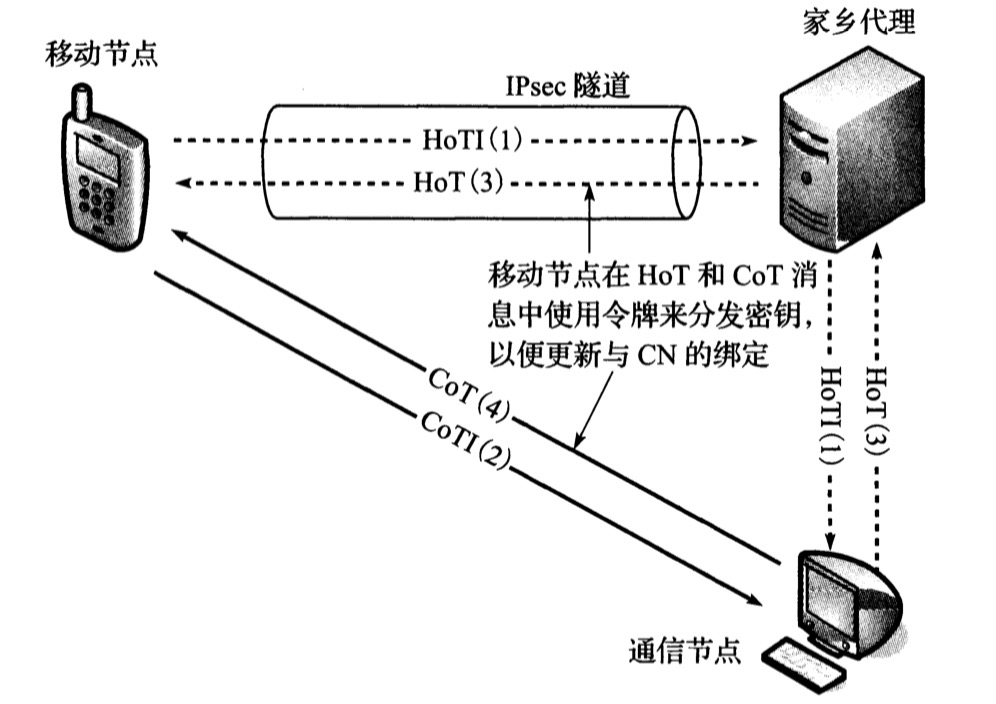HoT消息经由HA发送到MN，消息中包含称为令牌的随机字符串, MN使用它形成一个加密密钥，这个密钥被用于生成发送给CN的经过认证的绑定更新。

IP数据报的主机处理

强主机模式中，只有当"目的IP地址字段中包含的IP地址"与"数据报到达的接口配置的IP地址"匹配时，才同意把将数据报交付本地协议栈。

弱主机模式中，"数据报携带的目的地址"与"它到达的任何接口的任何本地地址"匹配，无论它到达哪个网络接口，它都会被接收的协议栈处理。

强主机模式中，只有在"数据报的源IP地址"所对应的网络接口才可以发送数据报。

弱主机模式中，无论哪个网络接口都可以发送数据报。

1. 该算法定义了一个源地址的候选集合CS(D)，基于一个特点的目的地址D(除去任播、组播和未指定地址)。

2. 符号R(A)表示地址A在集合CS(D)中的等级，A比B的等级高，则R(A)>R(B)，意味着优先选用A作为到达地址D的源地址。

3. R(A)*>R(B)表示在CS(D)中为A分配一个比B更高的等级。

4. I(D)表示选择到达目的D的接口(通过最长匹配前缀转发算法获取)。

5. @i是分配给接口i的地址集合。

6. 如果A是一个临时地址，T(A)为布尔值true，否则为false。

7. Q(D)表示目的地D选择一个最高等级的源地址，如果Q(D)=φ(空)，可能无法为目的地D确定源地址。

1. 优先选择相同地址：if A = D, R(A) *> R(B);if B = D, R(B) *> R(A).

2. 优先选择适当范围：if S(A) < S(B) and S(A) < S(D), R(B) *> R(A) else R(A) *> R(B);if S(B) < S(A) and S(B) < S(D), R(A) *> R(B) else R(B) *> R(A).

3. 避免过期地址：if S(A)=S(B), {if ∧(A)<∧(B), R(B)*>R(A) else R(A)*>R(B)}.

4. 优先选择家乡地址：if H(A) and C(A) and ┐(C(B) and H(B)), R(A) *> R(B);if H(B) and C(B) and ┐(C(B) and H(A)), R(B) *> R(A);if (H(A) and ┐C(A)) and (┐H(B) and C(B)), R(A) *> R(B);if (H(B) and ┐C(B)) and (┐H(A) and C(A)), R(B) *> R(A).

5. 优先选择输出接口：if A ∈ @(I(D)) and B ∈ @(I(D)), R(A) *> R(B);if B ∈ @(I(D)) and A ∈ @(I(D)), R(B) *> R(A).

6. 优先选择匹配标签：if L(A) = L(D) and L(B) ≠ L(D), R(A) *> R(B);if L(B) = L(D) and L(A) ≠ L(D), R(B) *> R(A).

7. 优先选则非临时地址：if T(B) and ┐T(A), R(A) *> R(B);if T(A) and ┐T(B), R(B) *> R(A).

8. 使用最长匹配前缀：if CPL(A,D) > CPL(B,D), R(A) *> R(B);if CPL(B,D) > CPL(A,D), R(B) *> R(A).

1. Q(D)是为目的地D选择的源地址

2. 如果目的地B不可到达，则U(B)为布尔值true

3. E(A)表示采用某些"封装传输"可到达目的地A

4. 集合SD(S)采用与前面的成对元素A和B相同的结构

1. 避免不可用的目的地：if U(B) or Q(B) = φ, R(A) *> R(B);if U(A) or Q(A) = φ, R(B) *> R(A).

2. 优先选择匹配范围：if S(A) = S(Q(A)) and S(B) ≠ S(Q(B)), R(A) *> R(B);if S(B) = S(Q(B)) and S(A) ≠ S(Q(A)), R(B) *> R(A).

3. 避免过期地址：if ∧(Q(A)) < ∧(Q(B)), R(B) *> R(A);if ∧(Q(B)) < ∧(Q(A)), R(A) *> R(B).

4. 优先选择家乡地址：if H(Q(A)) and C(Q(A)) and ┐(C(Q(B)) and H(Q(B))), R(A) *> R(B);if (Q(B)) and C(Q(B)) and ┐(C(Q(A)) and H(Q(A))), R(B) *> R(A);if (H(Q(A)) and ┐C(Q(A))) and (┐H(Q(B)) and C(Q(B))), R(A) *> R(B);if (H(Q(B)) and ┐C(Q(B))) and (┐H(Q(A)) and C(Q(A))), R(B) *> R(A).

5. 优先选择匹配标签：if L(Q(A)) = L(A) and L(Q(B)) ≠ L(B), R(A) *> R(B);if L(Q(A)) ≠ L(A) and L(Q(B)) = L(B), R(B) *> R(A).

6. 优先选择更高优先级：if P(A) > P(B), R(A) *> R(B);if P(A) < P(B), R(B) *> R(A).

7. 优先选择本地传输：if E(A) and ┐E(B), R(B) *> R(A);if E(B) and ┐E(A), R(A) *> R(B).

8. 优先选择更小范围：if S(A) < S(B), R(A) *> R(B) else R(B) *> R(A).

9. 使用最长匹配前缀：if CPL(A,Q(A)) > CPL(B,Q(B)), R(A) *> R(B);if CPL(A,Q(A)) < CPL(B,Q(B)), R(B) *> R(A).

10. 否则，保持等级顺序不变44 0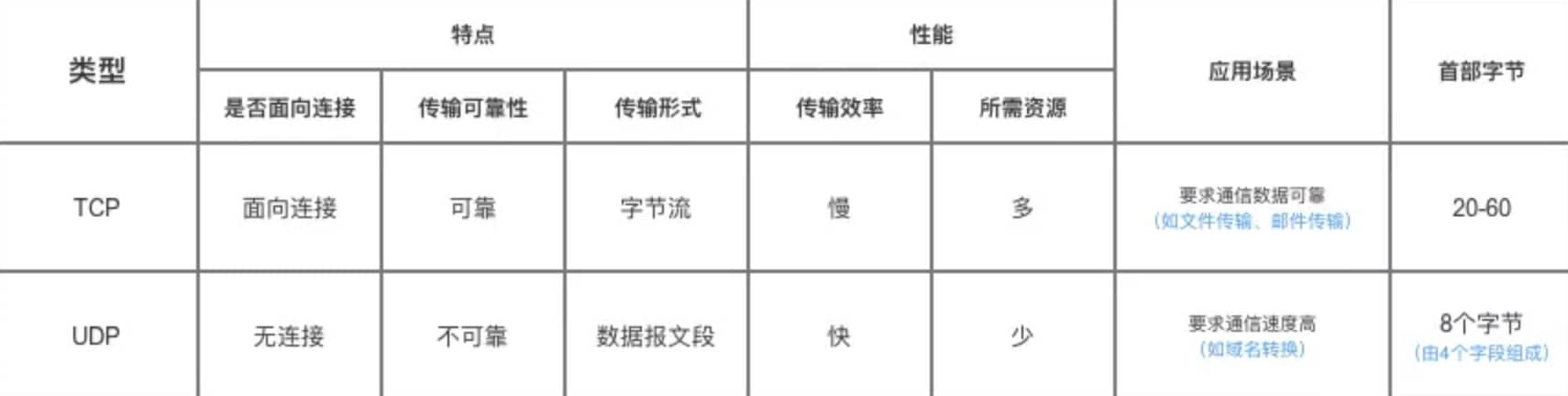《我要进大厂》- 计算机网络夺命连环20问，你能坚持到第几问？（应用层协议 | TCP三次握手、四次挥手 | TCP可靠传输 | Cookie&Session）（下）
《我要进大厂》- 计算机网络夺命连环20问，你能坚持到第几问？（应用层协议 | TCP三次握手、四次挥手 | TCP可靠传输 | Cookie&Session）
74 0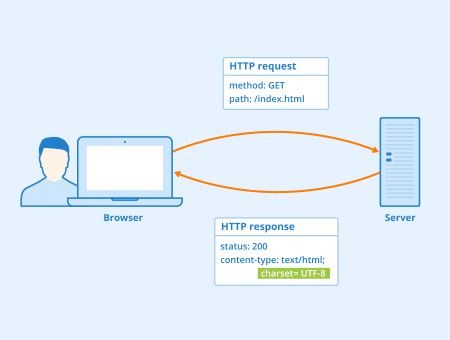《我要进大厂》- 计算机网络夺命连环20问，你能坚持到第几问？（应用层协议 | TCP三次握手、四次挥手 | TCP可靠传输 | Cookie&Session）（上）
《我要进大厂》- 计算机网络夺命连环20问，你能坚持到第几问？（应用层协议 | TCP三次握手、四次挥手 | TCP可靠传输 | Cookie&Session）
52 0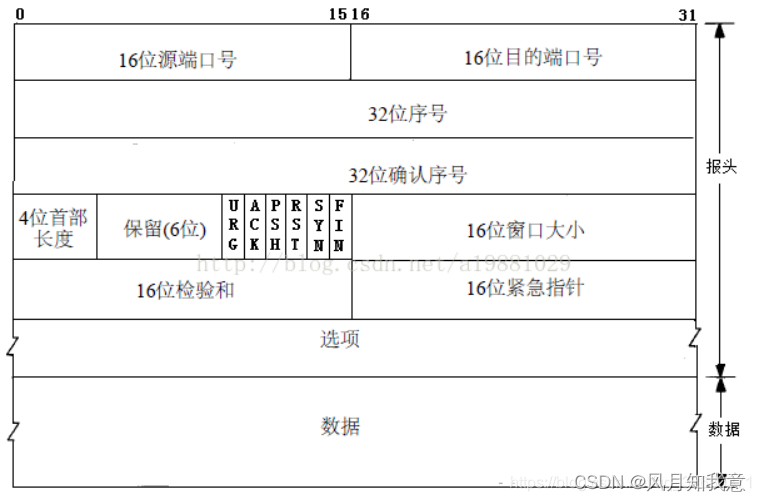TCP概述 TCP是一种面向连接的协议，在发送数据前通信双方必须在彼此间建立一条连接 所谓的连接其实就是客户端和服务器的内存里保存一份关于对方的信息，如IP地址、端口 TCP是一种字节流，它会处理IP层的丢包、重复以及错误问题 在建立连接的过程中，双方交换的一些参数可以放到TCP的头部 总结 ：TCP提供了一种可靠、面向连接、字节流、传输层的服务，采用三次握手建立一个连接，四次挥手关闭一个连接
63 0java实现TCP协议文件传输
java实现TCP协议文件传输
56 0Http协议与TCP协议简单理解
Http协议与TCP协议简单理解
66 02022-渗透测试-信息收集-Metasploit(基于TCP协议)
2022-渗透测试-信息收集-Metasploit(基于TCP协议)
33 0

Linux网络进阶 - TCP/IP协议及OSI七层模型141275

TCP/IP 网络基础9974

Go语言核心编程 - 面向对象、文件、单元测试、反射、TCP编程1314162

TCP Cluster for mqtt技术实施方案